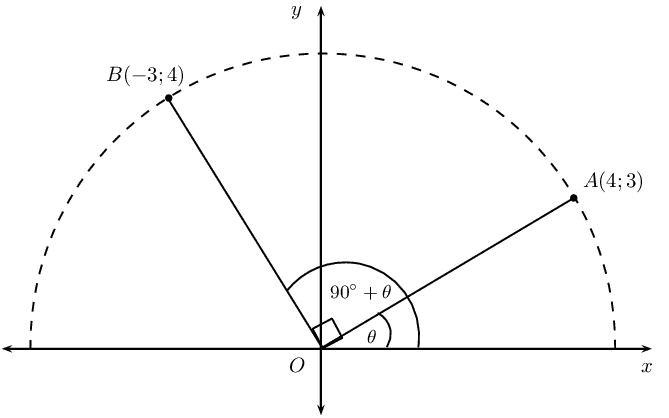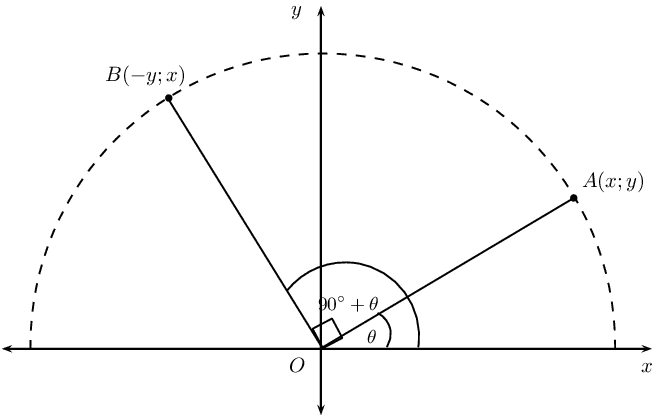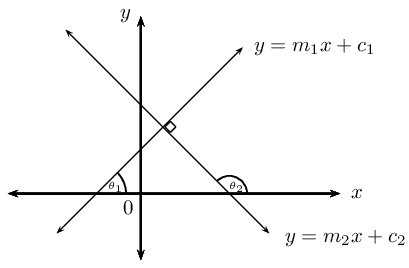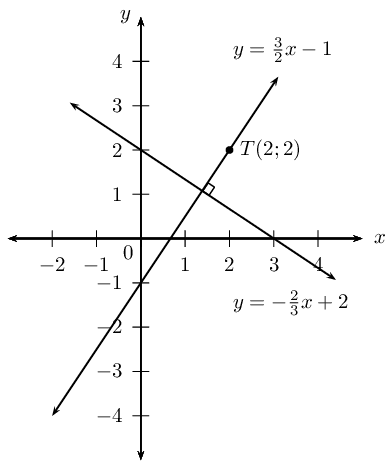Home Practice
For learners and parents For teachers and schools
Textbooks
Full catalogue
Pricing SupportLog in

We think you are located in United States. Is this correct?

# 4.5 Perpendicular lines

## Perpendicular lines

1. Draw a sketch of the line passing through the points $$A(-2;-3)$$ and $$B(2;5)$$ and the line passing through the points $$C(-1;\frac{1}{2})$$ and $$D(4;-2)$$.
2. Label and measure $$\alpha$$ and $$\beta$$, the angles of inclination of straight lines $$AB$$ and $$CD$$ respectively.
3. Label and measure $$\theta$$, the angle between the lines $$AB$$ and $$CD$$.
4. Describe the relationship between the lines $$AB$$ and $$CD$$.
5. “$$\theta$$ is a reflex angle, therefore $$AB \perp CD$$.” Is this a true statement? If not, provide a correct statement.
6. Determine the equation of the straight line $$AB$$ and the line $$CD$$.
7. Use your calculator to determine $$\tan \alpha \times \tan \beta$$.
8. Determine $$m_{AB} \times m_{CD}$$.
9. What do you notice about these products?
10. Complete the sentence: if two lines are $$\ldots \ldots$$ to each other, then the product of their $$\ldots \ldots$$ is equal $$\ldots \ldots$$
11. Complete the sentence: if the gradient of a straight line is equal to the negative $$\ldots \ldots$$ of the gradient of another straight line, then the two lines are $$\ldots \ldots$$

Deriving the formula: $$m_1 \times m_2 = -1$$Consider the point $$A(4;3)$$ on the Cartesian plane with an angle of inclination $$A\hat{O}X = \theta$$. Rotate through an angle of $$\text{90}\text{°}$$ and place point $$B$$ at $$(-3;4)$$ so that we have the angle of inclination $$B\hat{O}X = \text{90}\text{°}+ \theta$$.

We determine the gradient of $$OA$$: \begin{align*} m_{OA} &= \frac{y_2 - y_1}{x_2 - x_1} \\ &= \frac{3 - 0}{4 - 0} \\ &= \frac{3}{4} \end{align*}

And determine the gradient of $$OB$$: \begin{align*} m_{OB} &= \frac{y_2 - y_1}{x_2 - x_1} \\ &= \frac{4 - 0}{-3 - 0} \\ &= \frac{4}{-3} \end{align*}

By rotating through an angle of $$\text{90}\text{°}$$ we know that $$OB \perp OA$$: \begin{align*} m_{OA} \times m_{OB} &= \frac{3}{4} \times \frac{4}{-3} \\ &= -1 \end{align*}

We can also write that

$m_{OA} = -\frac{1}{m_{OB}}$If we have the general point $$A(x;y)$$ with an angle of inclination $$A\hat{O}X = \theta$$ and point $$B(-y;x)$$ such that $$B\hat{O}X = \text{90}\text{°}+ \theta$$, then we know that

\begin{align*} m_{OA} &= \frac{y}{x} \\ m_{OB} &= -\frac{x}{y} \\ \therefore m_{OA} \times m_{OB}&= \frac{y}{x} \times -\frac{x}{y} \\ &= -1 \end{align*}

Another method of determining the equation of a straight line is to be given a point on the line, $$\left({x}_{1};{y}_{1}\right)$$, and the equation of a line which is perpendicular to the unknown line. Let the equation of the unknown line be $$y = m_1x + c_1$$ and the equation of the given line be $$y = m_2x + c_2$$.If the two lines are perpendicular then

$m_1 \times m_2 = -1$

Note: this rule does not apply to vertical or horizontal lines.

When determining the gradient of a line using the coefficient of $$x$$, make sure the given equation is written in the gradient–intercept (standard) form $$y = mx + c$$. Then we know that $m_1 = -\frac{1}{m_2}$

Substitute the value of $$m_1$$ and the given point $$\left({x}_{1};{y}_{1}\right)$$, into the gradient–intercept form of the straight line equation $$y-y_1 = m(x-x_1)$$ and determine the equation of the unknown line.

## Worked example 13: Perpendicular lines

Determine the equation of the straight line passing through the point $$T(2;2)$$ and perpendicular to the line $$3y + 2x - 6 = 0$$.

### Write the equation in standard form

Let the gradient of the unknown line be $$m_1$$ and the given gradient be $$m_2$$. We write the given equation in gradient–intercept form and determine the value of $$m_2$$.

\begin{align*} 3y + 2x - 6 &= 0 \\ 3y & = -2x + 6 \\ y & = -\frac{2}{3}x + 2\\ \therefore m_2 & = -\frac{2}{3} \end{align*}

We know that the two lines are perpendicular, therefore $$m_1 \times m_2 = -1$$. Therefore $$m_1 = \frac{3}{2}$$.

### Write down the gradient–point form of the straight line equation

$y - y_1 = m(x - x_1)$

Substitute $$m_1 = \frac{3}{2}$$.

$y - y_1 = \frac{3}{2}(x - x_1)$

Substitute the given point $$T(2;2)$$.

\begin{align*} y - 2 & = \frac{3}{2}(x - 2) \\ y - 2& = \frac{3}{2}x - 3\\ y & = \frac{3}{2}x - 1 \end{align*}A sketch was not required, but it is useful for checking the answer.

The equation of the straight line is $$y = \frac{3}{2}x - 1$$.

temp text

## Worked example 14: Perpendicular lines

Determine the equation of the straight line passing through the point $$(2;\frac{1}{3})$$ and perpendicular to the line with an angle of inclination of $$\text{71,57}$$$$\text{°}$$.

### Use the given angle of inclination to determine gradient

Let the gradient of the unknown line be $$m_1$$ and let the given gradient be $$m_2$$.

\begin{align*} m_{2} & = \tan \theta \\ & = \tan \text{71,57}\text{°} \\ & = \text{3,0} \end{align*}

Since we are given that the two lines are perpendicular,

\begin{align*} m_{1} \times m_{2} &= -1 \\ \therefore m_{1} &= - \frac{1}{3} \end{align*}

### Write down the gradient–point form of the straight line equation

$y - y_1 = m(x - x_1)$

Substitute the gradient $$m_{1} = -\frac{1}{3}$$.

$y - y_1 = -\frac{1}{3}(x - x_1)$

Substitute the given point $$(2;\frac{1}{3})$$.

\begin{align*} y - \left(\frac{1}{3}\right) & = -\frac{1}{3}(x - 2) \\ y -\frac{1}{3} & = -\frac{1}{3}x + \frac{2}{3}\\ y & = -\frac{1}{3}x + 1 \end{align*}

The equation of the straight line is $$y = -\frac{1}{3}x + 1$$.

## Perpendicular lines

Textbook Exercise 4.8

Calculate whether or not the following two lines are perpendicular:

$$y - 1 = 4x$$ and $$4y + x + 2 = 0$$

\begin{align*} y - 1 &= 4x \\ y &= 4x + 1 \\ \therefore m_1 &= 4\\ 4y + x + 2 &= 0 \\ 4y &= - x - 2 \\ y &= - \frac{1}{4}x - \frac{1}{2} \\ \therefore m_2 &= -\frac{1}{4} \\ \therefore m_1 \times m_2&= -\frac{1}{4} \times 4 \\ &= -1 \end{align*}

$$\therefore$$Perpendicular lines.

$$10x = 5y - 1$$ and $$5y - x - 10 = 0$$

\begin{align*} 10x &= 5y - 1 \\ 10x + 1 &= 5y \\ 2x + \frac{1}{5} &= y \\ \therefore m_1 &= 2 \\ 5y - x - 10 &= 0 \\ 5y &= - x + 10 \\ y &= - \frac{1}{5}x + 2 \\ \therefore m_2 &= -\frac{1}{5} \\ \therefore m_1 \times m_2&= -\frac{1}{5} \times 2 \\ &\ne -1 \end{align*}

$$\therefore$$Not perpendicular lines.

$$x = y - 5$$ and the line passing through $$(-1;\frac{5}{4})$$ and $$(3;-\frac{11}{4})$$

\begin{align*} x &= y - 5 \\ x + 5 &= y \\ \therefore m_1 &= 1 \\ m_2 &= \frac{y_2 - y_1}{x_2 - x_1} \\ &= \frac{-\frac{11}{4} -\frac{5}{4}}{3 + 1} \\ &= \frac{-4}{4} \\ \therefore m_2 &= -1 \\ \therefore m_1 \times m_2&= -1 \times 1 \\ &= -1 \end{align*}

$$\therefore$$Perpendicular lines.

$$y = 2$$ and $$x = 1$$

Perpendicular: horizontal and vertical lines.

$$\frac{y}{3} = x$$ and $$3y + x = 9$$

\begin{align*} \frac{y}{3} &= x \\ y &= 3x \\ \therefore m_1 &= 3 \\ 3y + x &= 9 \\ 3y &= -x + 9 \\ y &= -\frac{1}{3}x + 3 \\ \therefore m_2 &= -\frac{1}{3} \\ \therefore m_1 \times m_2&= -\frac{1}{3} \times 3 \\ &= -1 \end{align*}

$$\therefore$$Perpendicular lines.

$$1 - 2x = y$$ and the line passing through $$(2;-1)$$ and $$(-1;5)$$

\begin{align*} 1 -2x &= y \\ \therefore m_1 &= -2 \\ m_2 &= \frac{y_2 - y_1}{x_2 - x_1} \\ &= \frac{5 + 1}{-1-2} \\ &= \frac{6}{-3} \\ \therefore m_2 &= -2 \\ \therefore m_1 \times m_2&= -2 \times -2 \\ &\ne -1 \end{align*}

$$\therefore$$Not perpendicular lines.

$$y = x + 2$$ and $$2y + 1 = 2x$$

\begin{align*} y &= x + 2 \\ \therefore m_1 &= 1 \\ 2y + 1 &= 2x \\ 2y &= 2x - 1 \\ y &= x - \frac{1}{2} \\ \therefore m_2 &= 1 \\ \therefore m_1 \times m_2&= 1 \times 1 \\ &\ne -1 \end{align*}

$$\therefore$$Not perpendicular lines.

Determine the equation of the straight line that passes through the point $$(-2;-4)$$ and is perpendicular to the line $$y + 2x = 1$$.

\begin{align*} y &= -2x + 1 \\ \therefore m_1 &= -2 \\ \text{For } \perp: m_1 \times m_2 &= -1 \\ -2 \times m_2 &= -1 \\ \therefore m_2 &= \frac{1}{2} \\ y &= mx + c \\ y &= \frac{1}{2}x + c \\ \text{Subst. } (-2;-4): \quad - 4 &= \frac{1}{2}(-2) + c \\ -4 &= -1 + c \\ \therefore c &= -3 \\ \therefore y &= \frac{1}{2}x - 3 \end{align*}

Determine the equation of the straight line that passes through the point $$(2;-7)$$ and is perpendicular to the line $$5y - x = 0$$.

\begin{align*} 5y - x &= 0 \\ 5y &= x \\ y &= \frac{1}{5}x \\ \therefore m_1 &= \frac{1}{5} \\ \text{For } \perp: m_1 \times m_2 &= -1 \\ \frac{1}{5} \times m_2 &= -1 \\ \therefore m_2 &= -5 \\ y &= mx + c \\ y &= -5x + c \\ \text{Subst. } (2;7): \quad -7 &= -5(2) + c \\ -7 &= -10 + c \\ \therefore c &= 3 \\ \therefore y &= -5x + 3 \end{align*}

Determine the equation of the straight line that passes through the point $$(3;-1)$$ and is perpendicular to the line with angle of inclination $$\theta = \text{135}\text{°}$$.

\begin{align*} \theta &= \text{135}\text{°} \\ \therefore m &= \tan \theta \\ &= \tan \text{135}\text{°} \\ \therefore m_{1} &= -1 \\ \text{For } \perp: m_{1} \times m_{2} &= -1 \\ -1 \times m_{2} &= -1 \\ \therefore m_{2} &= 1 \\ y &= mx + c \\ y &= x + c \\ \text{Subst. } (3;-1): \quad -1 &= (3) + c \\ \therefore c &= -4 \\ \therefore y &= x - 4 \end{align*}

Determine the equation of the straight line that passes through the point $$(-2;\frac{2}{5})$$ and is perpendicular to the line $$y = \frac{4}{3}$$.

$$x = -2$$